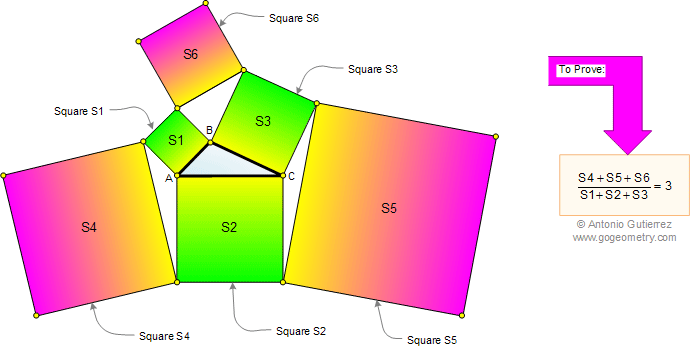Problem 520: Triangle, Six Squares, Areas, Ratio. Level: High School, College, SAT Prep. The drawing shows a triangle ABC with the squares of areas S1, S2, S3, S4, S5, and S6. Prove that the ratio between the sum of areas S4+S5+S6 , and the sum of areas S1+S2+S3 is equal to 3. See also: Artwork of problem 520, Warp Speed, Hyperdrive.Recent Additions